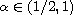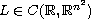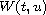Electron. J. Diff. Equ., Vol. 2016 (2016), No. 40, pp. 1-13.

### Existence of solutions to fractional Hamiltonian systems with combined nonlinearities Ziheng Zhang, Rong Yuan

Abstract:
This article concerns the existence of solutions for the fractional Hamiltonian systemwhere,is a symmetric and positive definite matrix. The novelty of this article is that ifand the nonlinearityinvolves a combination of superquadratic and subquadratic terms, the Hamiltonian system possesses at least two nontrivial solutions.

Submitted November 11, 2015. Published January 27, 2016.
Math Subject Classifications: 34C37, 35A15, 35B38.
Key Words: Fractional Hamiltonian systems; critical point; variational methods; mountain pass theorem.

Show me the PDF file (269 KB), TEX file for this article.Ziheng Zhang Department of Mathematics Tianjin Polytechnic University Tianjin 300387, China email: zhzh@mail.bnu.edu.cn Rong Yuan Department of Mathematical Sciences Beijing Normal University Beijing 100875, China email: ryuan@bnu.edu.cn